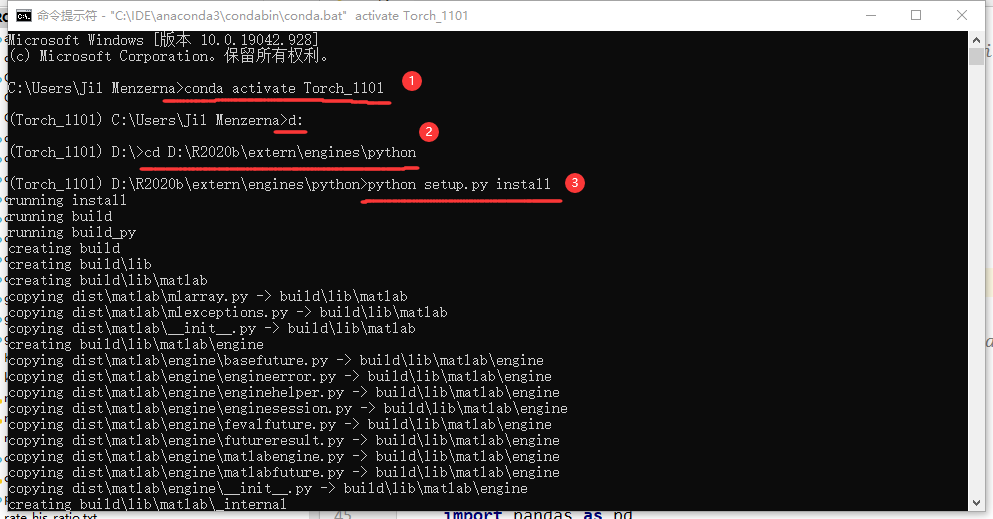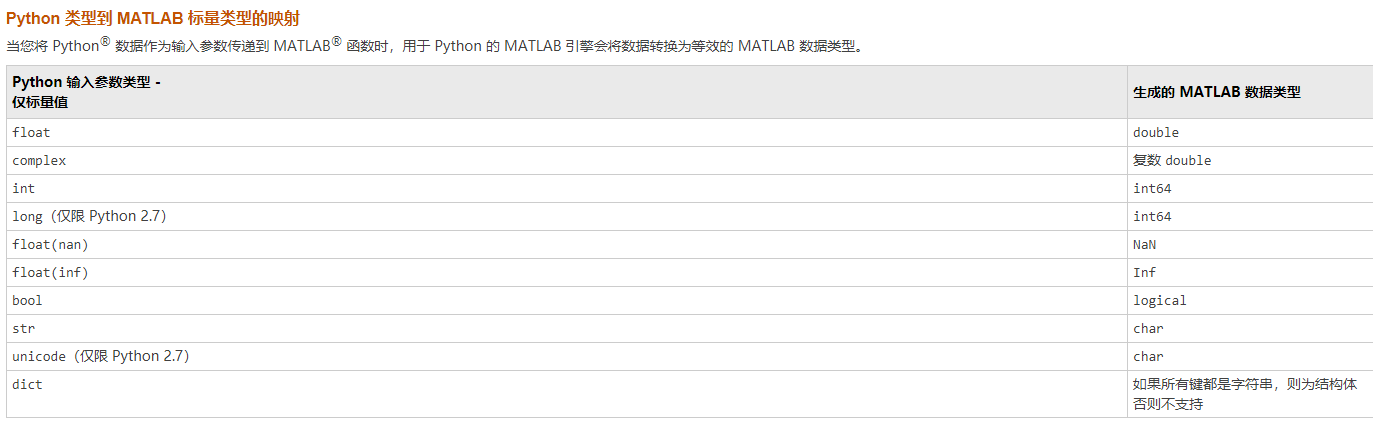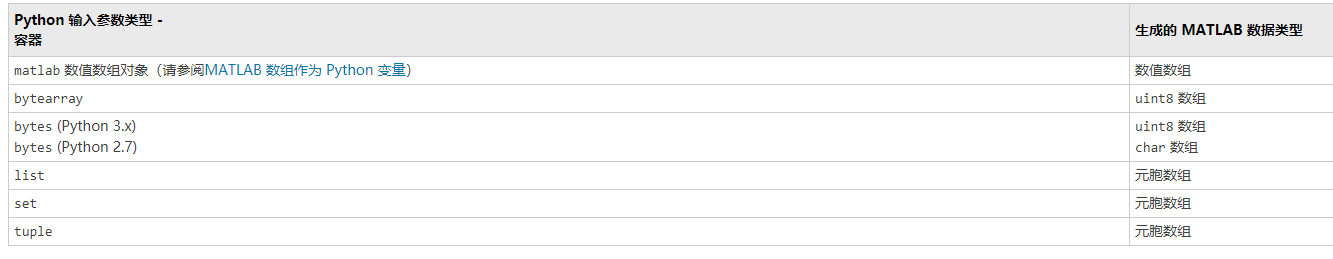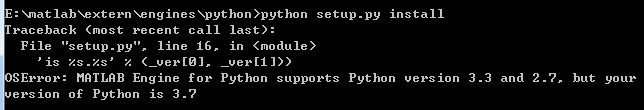• python调用matlab函数1、matlab中python外部接口（API）的安装2、matlab.egine模块3、从Python传递数值到Matlab 1、matlab中python外部接口（API）的安装 第一次使用Matlab需要先安装一下python的API。 路径为：…\...
python调用matlab函数1、matlab中python外部接口（API）的安装2、matlab.egine模块3、从Python传递数值到Matlab
1、matlab中python外部接口（API）的安装
第一次使用Matlab需要先安装一下python的API。
路径为：…\Matlab2019a\extern\engines\python\setup.py
需注意matlab版本与其所支持的python版本，如 Matlab2018b支持python 3.4 3.5 3.6，2019a支持到3.7安装如下三步：
1、激活需要安装matlab模块的环境
2、切换到matlab该文件路径下路径
3、调用python setup.py install，进行安装2、matlab.egine模块
使用python调用matlab函数（function）：
例子：想使用python调用matlab函数，且函数内嵌套了matlab的CVX包，用于解凸优化问题。
新建一个python脚本：test.py
import matlab.egine
def CVX_Matlab(h,t):

eng = matlab.engine.start_matlab()  # 启动matlab,此时可以调用matlab内置函数
a = eng.CVX_Matlab(h,t)
print(a)

if __name__ == '__main__':
h = [2.1062e-7, 1.8093e-7,1.502e-7]
t = 6.5
CVX_Matlab(h,t)

在matlab中，编写好你需要使用的函数 CVX.m
function [optValue, Slist] = CVX_Matlab(g,t)

% g的类型为'cell'  g=[{2.1062e-7}, {1.8093e-7},{1.502e-7}]                   % g为python以列表形式传入的信道增益

Slist = [];
T = [];
optValue = [];

t = t/1000;                                      % NOMA通讯时间 ms级别,换算成s
n = 3;                                      % 边缘服务器个数
S = 15e3;                                   % 总任务量
y = [10e6, 15e6,20e6];                     % 边缘服务器计算速率
W = 8e6;                                    % 带宽
uL = 1e6;                                   % 本地计算速率
P = 5;                                      % 本地NOMA发送功率
n0 = 1e-12;                                 % 高斯白噪声功率谱密度

cvx_begin
cvx_precision best
variable Sm(n)

minimize(max([(S-(Sm(1)+Sm(2)+Sm(3))*S) / uL,t/1 + Sm(1)*S/y(1),t + Sm(2)*S/y(2),t + Sm(3)*S/y(3)] ))
subject to
Sm(1)+Sm(2)+Sm(3) <= 1
Sm(1) >= 0
Sm(2) >= 0
Sm(3) >= 0
SUM = (1/g{1})*power(2,(1/t)*(1/W)*(Sm(1)+Sm(2)+Sm(3))*S)+(1/g{2}-1/g{1})*power(2,(1/t)*(1/W)*(Sm(2)+Sm(3))*S)+(1/g{3}-1/g{2})*power(2,(1/t)*(1/W)*(Sm(3))*S)
P >= W*n0*SUM -W*n0/g{3}
cvx_end

A = (S-(Sm(1)+Sm(2)+Sm(3))*S) / uL;
B = t/1 + Sm(1)*S/y(1);
C = t + Sm(2)*S/y(2);
D = t + Sm(3)*S/y(3);

Slist = [Slist,Sm];
T = [T,t];
optValue = [optValue,cvx_optval*1000]

3、从Python传递数值到Matlab
Python 类型到 MATLAB 标量类型的映射Python 容器到 MATLAB 数组类型的映射其中元胞数组（cell类型），需使用 {} 进行索引访问。
cell的操作

cell的创建：

（1）跟一般创建矩阵一样，直接使用C = {A B D E}这种形式，不过这里把"[]“改成了”{}"
（2）使用cell创建cell,C= {C1 C2}, 是1×2 cell 数组
使用C3 = [C1 C2],是使用C1和C2的内容来创建
（3）使用cell函数，A=cell(2,2),创建一个空的2x2的cell矩阵.

cell的访问

X= C(s)使用这种"()"形式的返回的是cell类
X = C{s}使用这种"{}"形式的返回的是cell中的内容
其他的基本上与通常的数组.矩阵访问一样了
A{1,1}(2,3:end)访问的是A中的第一个cell里面内容的第2行的第三个元素以后的所有元素
A{1,3}.name访问的是A中的A{1,3}cell里面结构的name值
cell的图形化显示使用命令cellplot(A)即可如B＝{[1；2]}，这就定义了一个cell型的变量B，里面保存的是一个矩阵[1；2]，要引用里面的数据只要在大括号里写上对应的下标即可，如B{1}。

下面我们给B再加个元素，我们可以这样定义B（2）＝{‘hello’}。也可以利用引用的方法定义B{2}＝’hello’。第二种方法比第一种方法更方便所以更常用。
这样B就有两个元素了，B（1）是{[1；2]}，B（2）是{‘hello’}，每个元素都是cell型的（注意和B{1}、B{2}的区别，B{1}是[1；2]，B{2}是’hello’）。


展开全文• 遇到求解西尔维斯特方程时不会，然后查资料也看不懂，结果发现matlab有直接的函数可以调用，无奈就只能用Python调用matlab函数。 安装matlab包 首先要安装matlab这个包，可以使用matlab里带的setup.py这个脚本。 cd ...
遇到求解西尔维斯特方程时不会，然后查资料也看不懂，结果发现matlab有直接的函数可以调用，无奈就只能用Python调用matlab函数。
安装matlab包
首先要安装matlab这个包，可以使用matlab里带的setup.py这个脚本。
cd "MatlabRoot\extern\engines\python"
python setup.py install

MatlabRoot是你自己matlab的路径，以上是windows的命令，Linux命令用斜杠。
注意事项：
1.windows可能需要管理员权限。
2.matlab和Python的版本有对应要求，比如我的matlab用2014b，Python就需要3.3或2.7。
即使修改了setup中的版本检查，安装完在实际使用中还是会出问题。3.安装完以后会在AnacondaRoot/Lib/site-packages中出现一个matlab包，这时候在工程中还是无法import，需要在工程环境或虚拟环境中再添加一下。
调用matlab的API
这里就不写了，这个博文有着详细地描述。
我只是达成了自己调用matlab函数的需求，所以只是简单使用，大家有需要、有兴趣的可以再实践和讨论。


展开全文• 这是我发现的：pymat使用matlab引擎（libeng）进行通信的Matlab底层接口（基本上是matlab随附的库）。该模块必须编译并与libeng链接。http://pymat.sourceforge.netpymat2脓垫发育的持续时间较短。似乎可以在Wind...
我知道这是一个老问题，已经得到答复。但是我一直在寻找相同的东西（对于Mac），发现有很多选择与matlab交互的方法和成熟度不同。这是我发现的：pymat使用matlab引擎（libeng）进行通信的Matlab底层接口（基本上是matlab随附的库）。该模块必须编译并与libeng链接。http://pymat.sourceforge.netpymat2脓垫发育的持续时间较短。似乎可以在Windows（包括64位），Linux和Mac（经过一些更改）上工作。https://code.google.com/p/pymat2/包裹一个高级接口，它也是一个模块，需要编译和链接 libeng。它将Matlab函数公开给python，因此您可以做一些有趣的事情，例如mlab.plot(x, y, 'o')http://mlabwrap.sourceforge.net玛拉mlabwrap的重新包装工作。基本上，它取代了C ++代码，对在“libeng”链接mlabwrap用蟒模块（matlabpipe），其与通过管道MATLAB连通。这样做的主要优点是不需要任何形式的编译。不幸的是，该软件包当前存在一些错误，并且似乎根本无法在Mac上运行。我报告了其中一些，但最终放弃了。另外，如果您必须进入源代码，请做好准备，以应对大量的骗局和大量丑陋的骇客；-)如果这变得更加成熟，则可能是最佳选择之一。https://github.com/ewiger/mlabpymatlab较新的软件包（2010）也可以通过与Matlab进行交互libeng。与其他软件包不同，此软件包通过ctypes加载引擎库，因此无需编译。它不是没有缺陷，但仍然可以维护，我发现的（特定于64bit Mac的）问题应该很容易解决。http://pymatlab.sourceforge.netpython-matlab-bridge还有一个仍在积极维护的更新程序包。通过某种套接字与Matlab通信。不幸的是，公开的功能有些限制。我不知道如何调用以结构为参数的函数。需要易于安装的zmq，pyzmq和IPython。http://arokem.github.io/python-matlab-bridge
展开全文• 0. 实验环境Ubuntu 16.04Matlab R2015b1. 借助于 mlab 库安装方法非常简单，只需一行命令 sudo pip install mlab 即可。import numpy as npfrom PIL import Imagefrom mlab.releases import latest_release as ...
0. 实验环境Ubuntu 16.04Matlab R2015b1. 借助于 mlab 库安装方法非常简单，只需一行命令 sudo pip install mlab 即可。import numpy as npfrom PIL import Imagefrom mlab.releases import latest_release as matlabimage = Image.open('1.jpg')image = np.array(image)h, w = image.shapeprint(image.shape) # (413, 295)在上面的代码中，我们先读入一个图片，然后将其转化为一个 Numpy 数组。接下来，假如我们想通过调用 MATLAB 的 imresize 函数来对这幅图像进行 4 倍上采样，那么我们要做的就是将这个 Numpy 数组传递到 MATLAB 中，然后调用相应的函数，最后再将结果返回到 Python 中。但是，MATLAB 并不支持将 Python 中的 Numpy 数组直接映射为矩阵，具体映射方式可参考 matlab.double(initializer=None, size=None, is_complex=False) 构造函数image = image.reshape(-1, 1)image = image.tolist()image = matlab.double(image)image = matlab.reshape(image, (h, w))resized_image = matlab.imresize(image, 4, 'bicubic')print(resized_image.shape) # (1652, 1180)如果我们想要调用自定义函数，比如下面这样的 m 文件。function c = add(a, b)c = a + b;end那么只需要传递相应的参数进去即可，这里 Python 中的浮点数会映射为 MATLAB 中的 double 类型。result = matlab.add(2.0, 3.0)print(result) # 5.0但是，目前在我这边发现 mlab 不支持 Python 3，安装后会提示 ImportError: No module named 'mlabwrap' 之类的错误，暂时还没找到解决方案。2. 借助于 MATLAB 自带的引擎 API首先，需要 /usr/local/MATLAB/R2015b/extern/engines/python，然后运行命令 sudo python setup.py install 即可。由于我的 MATLAB 版本还比较低，目前只支持到 Python 3.4，更高的版本则会报错 OSError: MATLAB Engine for Python supports Python version 2.7, 3.3 and 3.4, but your version of Python is 3.5。>>> import matlab.engine>>> eng = matlab.engine.start_matlab()>>> import numpy as np>>> image = np.random.randn(30, 30)>>> image.shape(30, 30)>>> resized_image = eng.imresize(image, 4, 'bicubic')Traceback (most recent call last):File "", line 1, in File "/usr/local/lib/python2.7/dist-packages/matlab/engine/matlabengine.py", line 79, in __call__out=_stdout, err=_stderr)TypeError: unsupported Python data type: numpy.ndarray>>> image = image.reshape(-1, 1)>>> image = image.tolist()>>> image = matlab.double(image)>>> image.reshape((30, 30))>>> resize_image = eng.imresize(image, 4, 'bicubic')>>> resize_image.size(120, 120)>>> eng.add(2.0, 3.0)5.0>>>用法和第一种类似，但是在我这边测试发现只能运行在交互模式下，直接运行对应的 py 文件则会报错。senius@HP:~/Downloads\$ python2 test1.pyTraceback (most recent call last):File "test1.py", line 3, in import matlab.engineFile "/usr/local/lib/python2.7/dist-packages/matlab/engine/__init__.py", line 60, in raise EnvironmentError('The installation of MATLAB Engine for Python is 'EnvironmentError: The installation of MATLAB Engine for Python is corrupted.Please reinstall it or contact MathWorks Technical Support for assistance.上面两种方法都只能进行一些简单的调用，而且还需要在 Python 和 MATLAB 之间进行数据转化，非常不方便，下面介绍的第三种方法则非常简单有效。3. 借助于 transplant 库sudo pip3 install transplant。Python 中的列表会转化为 MATLAB 中的元胞数组，Numpy 数组会转化为 MATLAB 中的矩阵，更多详细信息可参阅>>> import transplant>>> import numpy as np>>> matlab = transplant.Matlab(jvm=False, desktop=False)< M A T L A B (R) >Copyright 1984-2015 The MathWorks, Inc.R2015b (8.6.0.267246) 64-bit (glnxa64)August 20, 2015For online documentation, see http://www.mathworks.com/supportFor product information, visit www.mathworks.com.>>> image = np.random.randn(30, 30)>>> image.shape(30, 30)>>> resized_image = matlab.imresize(image, 4, 'bicubic')>>> resized_imagearray([[ 0.78619134,  0.7167187 ,  0.57147529, ..., -0.1314248 ,-0.19615895, -0.22226921],[ 0.69992414,  0.63668882,  0.50463212, ..., -0.10023177,-0.16053713, -0.18483134],[ 0.51579787,  0.46606682,  0.36253926, ..., -0.01897913,-0.07015085, -0.09065985],...,[ 0.27508006,  0.27579099,  0.28756401, ..., -0.70385557,-0.80006309, -0.84680474],[ 0.23260259,  0.23527929,  0.25076149, ..., -0.75840955,-0.80315767, -0.82872907],[ 0.21943022,  0.2228168 ,  0.2396492 , ..., -0.78075353,-0.80353953, -0.8200766 ]])>>> resized_image.shape(120, 120)>>>针对如下所示的多个自定义函数存在互相调用的复杂情况，transplant 也可以轻松胜任。比如，我们需要通过 Python 调用 NGmeet_DeNoising( N_Img, O_Img, nSig ) 这个函数，它有三个输入，N_Img 为长×宽×波段的三维噪声高光谱图像，O_Img  为对应的干净图像，而 nSig 为噪声等级。那么只需在 Python 中将两个 Numpy 数组和一个整数传给对应的函数即可。clean = np.load('GT_crop.npy')h, w, b = clean.shapesigma = 25noisy = clean + np.random.randn(h, w, b) * sigma / 255print(cal_psnr(clean, noisy)) # 20.1707c = matlab.NGmeet_DeNoising(255.0*noisy, 255.0*clean, sigma)print(c.shape, c.dtype) # (200, 200, 191) float64print(matlab.mpsnr(c/255, clean)) # 34.5712如果报如下的错误，则因为 par_nSig 是将 Python 中的 sigma=25 转化为了 MATLAB 中的 int64，而 int64 数据不能与 double 类数据相乘。而解决办法也很简单，double(par_nSig) 将其数据转化为 double 类型即可。
展开全文• Python调用matlab函数 #####绕坑避雷##### #####直达捷径##### #####含官方指导##### #####及所需软件下载地址##### #####内附实例#####
• python中启动matlab： engine = matlab.engine.start_matlab() 如果输入的变量X是表格式的数据（n*d），即 ndarray格式，需要进行转换后再输入： data_list= matlab.double(X.tolist())
• 项目信号处理和提取部分用到了matlab，...具体方法如下：1.python中安装mlab安装命令：python setup.py installMlab is a high-level python to Matlab bridge that lets Matlab look like a normal python library....
• Windows cmd打开，cd到：E:\MATLAB\R2016b\extern\engines\python输入（linux同理）：python setup.py install2.确保编辑器为本地环境，试验 import matlab.engine 是否可用3.matlab中写方程安装完成后，MATLAB写一...
• 最近一个工程中要调用一个matlab的大函数，实在是不想重新写，就简单的用python调用matlab。版本匹配我用的环境是Python 3.6.6 matlab 2018b，python的版本和matlab的版本必须匹配，在matlab 2018b中支持的python...
• 这是我发现的：pymat使用matlab引擎（libeng）进行通信的Matlab底层接口（基本上是matlab随附的库）。该模块必须编译并与libeng链接。http://pymat.sourceforge.netpymat2脓垫发育的持续时间较短。似乎可以在...
• 一个简单的界面，允许Python调用MATLAB函数。 与其他接口不同，MATLAB只需启动一次。 所有通信都是通过TCP服务器完成的（TCP服务器的信用归特温特D.Kroon大学所有）。 尚未在Windows上进行测试。 用法 初始化Matlab...
• 我对我的系统有类似的要求，这是我的解决方案：在MATLAB中有一个称为perl.m的函数，它允许你从MATLAB调用perl脚本。根据你使用的版本，它将位于某个地方C:\Program Files\MATLAB\R2008a\toolbox\matlab\general\perl...
• python调用matlab函数错误记录 官方教程 首先要保证的是机器上有python和matlab 注意！！！ python版本只支持2.7，3.4，3.5 python版本只支持2.7，3.4，3.5 步骤是： ①找到 MATLAB 文件夹的路径。启动 ...
• 一些比较古早的方法建议在python中安装mlab库来实现调用的，这个方法已经淘汰不用了，这里介绍比较新的方法。Step 1: install MATLAB Engine API for Python官网给出的方案：install MATLAB Engine API for Python ...
• 众所周知，Python凭借其众多的第三方模块，近年来被数据分析、机器学习、深度学习等爱好者所喜爱，最主要的是Python还是开源的。另一方面，MATLAB因其在...既然MATLABPython各有各的优势，那能不能将二者的优势...
• Python调用matlab函数( 使用matlab官方api,解决多维numpy矩阵输入问题)
• Is it possible to run MATLAB functions from within Python?I search the internet, I could only find PyMat. The bad thing is the compiled version only supports Python2.2 and I am using 2.6. So I tried t...
• Is it possible to run MATLAB functions from within Python?I search the internet, I could only find PyMat. The bad thing is the compiled version only supports Python2.2 and I am using 2.6. So I tried t...
• python调用MATLAB函数代码——从安装到使用 一、安装 首先附上官方教程 具体操作： 找到 MATLAB 文件夹的路径。启动 MATLAB，并在命令行窗口中键入 matlabroot。复制 matlabroot 所返回的路径。 在 macOS 或 ...
• I have a platform for python scripting, and I would like to call matlab functions inside. I have found several threads tackling the issue, among them those twoHowever, threads are either not recent, o...
• I have a platform for python scripting, and I would like to call matlab functions inside. I have found several threads tackling the issue, among them those twoHowever, threads are either not recent, o......

# python调用matlab函数python 订阅matlab 订阅